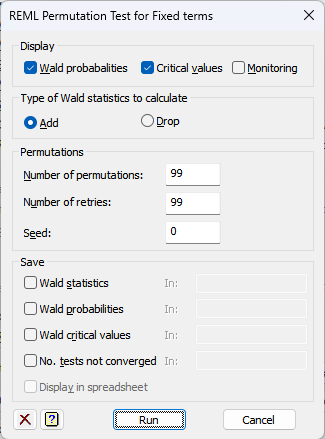1. Home
2. REML Permutation Test for Fixed Terms

# REML Permutation Test for Fixed Terms

This dialog allows you to assess the significance of the all the fixed terms in a REML analysis using a permutation test done by the VPERMTEST procedure.## Display

 Wald probabilities The wald probabilities calculated over the permutation set Critical values Critical values at the 5%, 1% and 0.1% significance levels for the Wald statistics Monitoring The progress of the permutation tests

## Type of Wald statistics to calculate

This option controls whether the significances of adding a term or dropping a term are calculated.

 Add The test statistics will be for adding the fixed terms. Drop The test statistics for the dropping fixed terms. Only those terms which are not marginal to others in the model can be dropped, e.g. with a model A + B + A.B, only A.B can be dropped as both A and B are marginal to A.B.

## Number of permutations

This option specifies the number of permutation samples that are performed, default 99 (as well as the “null” permutation where the data keep their original order).

## Number of retries

This option specifies the number of extra permutations that are performed when the REML model does not converge for a permutation, default 99. Increasing the value will give a more precise probability, but will slow down the analysis.

## Seed

Specifies the seed for the random number generator used to make the permutations; default 0 continues from the previous generation or (if none) initializes the seed automatically.

## Save

This lets you save results from the from the VPERMTEST analysis in Genstat data structures. After selecting the appropriate boxes, you need to type the names for the identifiers of the data structures into the corresponding In: fields.

 Wald statistics Pointer A pointer to a variates for all fixed terms. The variates contain the Wald statistics for all the successful permutations. The pointer suffixes are the names of the fixed terms. Wald probabilities Pointer A pointer to scalars giving the Wald probabilities for each fixed term. The pointer suffixes are the names of the fixed terms. Wald critical values Pointer A pointer to three variates for the 5%, 1% and 0.1% critical values for the Wald statistics. The variates contain a row for each fixed term. The variates have unit labels giving the names of the fixed terms. No. tests not converged Scalar The number of analyses of permuted data sets that did not converge.Clear Clear all fields and list boxes.Help Open the Help topic for this dialog.